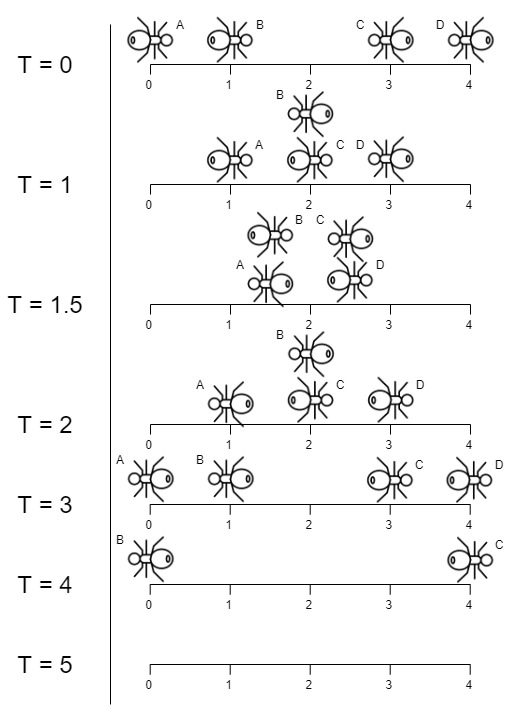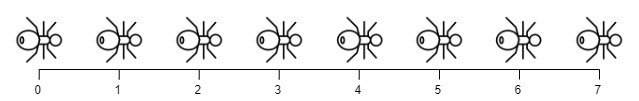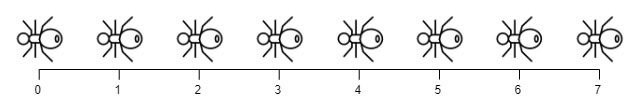##### Welcome to Subscribe On Youtube

Formatted question description: https://leetcode.ca/all/1503.html

# 1503. Last Moment Before All Ants Fall Out of a Plank (Medium)

We have a wooden plank of the length n units. Some ants are walking on the plank, each ant moves with speed 1 unit per second. Some of the ants move to the left, the other move to the right.

When two ants moving in two different directions meet at some point, they change their directions and continue moving again. Assume changing directions doesn't take any additional time.

When an ant reaches one end of the plank at a time t, it falls out of the plank imediately.

Given an integer n and two integer arrays left and right, the positions of the ants moving to the left and the right. Return the moment when the last ant(s) fall out of the plank.

Example 1:Input: n = 4, left = [4,3], right = [0,1]
Output: 4
Explanation: In the image above:
-The ant at index 0 is named A and going to the right.
-The ant at index 1 is named B and going to the right.
-The ant at index 3 is named C and going to the left.
-The ant at index 4 is named D and going to the left.
Note that the last moment when an ant was on the plank is t = 4 second, after that it falls imediately out of the plank. (i.e. We can say that at t = 4.0000000001, there is no ants on the plank).


Example 2:Input: n = 7, left = [], right = [0,1,2,3,4,5,6,7]
Output: 7
Explanation: All ants are going to the right, the ant at index 0 needs 7 seconds to fall.


Example 3:Input: n = 7, left = [0,1,2,3,4,5,6,7], right = []
Output: 7
Explanation: All ants are going to the left, the ant at index 7 needs 7 seconds to fall.


Example 4:

Input: n = 9, left = , right = 
Output: 5
Explanation: At t = 1 second, both ants will be at the same intial position but with different direction.


Example 5:

Input: n = 6, left = , right = 
Output: 6


Constraints:

• 1 <= n <= 10^4
• 0 <= left.length <= n + 1
• 0 <= left[i] <= n
• 0 <= right.length <= n + 1
• 0 <= right[i] <= n
• 1 <= left.length + right.length <= n + 1
• All values of left and right are unique, and each value can appear only in one of the two arrays.

Related Topics:
Array, Brainteaser

## Solution 1.

If two ants meet each other, changing their directions is the effectively the same as keeping them moving in their original directions.

// OJ: https://leetcode.com/problems/last-moment-before-all-ants-fall-out-of-a-plank/
// Time: O(N)
// Space: O(1)
class Solution {
public:
int getLastMoment(int n, vector<int>& left, vector<int>& right) {
int ans = 0;
for (int x : left) ans = max(ans, x);
for (int x : right) ans = max(ans, n - x);
return ans;
}
};

• class Solution {
public int getLastMoment(int n, int[] left, int[] right) {
Arrays.sort(left);
Arrays.sort(right);
int leftMost = -1, rightMost = -1;
if (left.length > 0)
leftMost = left[left.length - 1];
if (right.length > 0)
rightMost = right;
if (leftMost < 0 && rightMost < 0)
return 0;
if (leftMost < 0)
return n - rightMost;
else if (rightMost < 0)
return leftMost;
else
return Math.max(n - rightMost, leftMost);
}
}

############

class Solution {
public int getLastMoment(int n, int[] left, int[] right) {
int ans = 0;
for (int x : left) {
ans = Math.max(ans, x);
}
for (int x : right) {
ans = Math.max(ans, n - x);
}
return ans;
}
}

• // OJ: https://leetcode.com/problems/last-moment-before-all-ants-fall-out-of-a-plank/
// Time: O(N)
// Space: O(1)
class Solution {
public:
int getLastMoment(int n, vector<int>& left, vector<int>& right) {
int ans = 0;
for (int x : left) ans = max(ans, x);
for (int x : right) ans = max(ans, n - x);
return ans;
}
};

• class Solution:
def getLastMoment(self, n: int, left: List[int], right: List[int]) -> int:
ans = 0
for t in left:
ans = max(ans, t)
for t in right:
ans = max(ans, n - t)
return ans


• func getLastMoment(n int, left []int, right []int) (ans int) {
for _, x := range left {
ans = max(ans, x)
}
for _, x := range right {
ans = max(ans, n-x)
}
return
}

func max(a, b int) int {
if a > b {
return a
}
return b
}

• function getLastMoment(n: number, left: number[], right: number[]): number {
let ans = 0;
for (const x of left) {
ans = Math.max(ans, x);
}
for (const x of right) {
ans = Math.max(ans, n - x);
}
return ans;
}